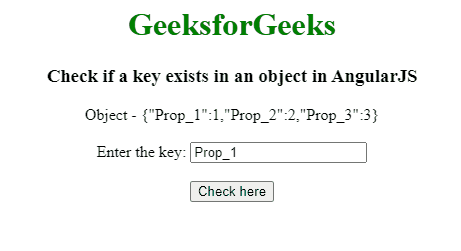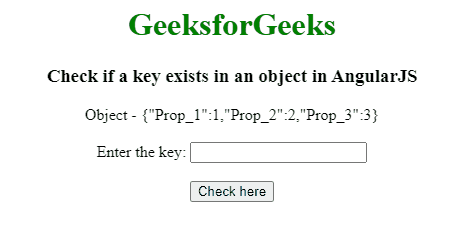Open In App

# How to check the existence of key in an object using AngularJS ?

Given an object containing a (key, value) pair and the task is to check whether a key exists in an object or not using AngularJS. In order to check the existence of a key in an object, we will create an object having properties in the form of a key: value pair. Define a temporary variable that will hold the initial key of an object. Create a function expression that will be utilized to check whether the temporary variable that holds an initial key, exists or not in the given object, by comparing them.

Approach: The approach is to use the in operator to check whether a key exists in an object or not. In the first example, the key “Prop_1” is input and it exists in the object. In the second example, the user can check which key they want to check for existence.

Example 1: In this example, the key “Prop_1” is input and checking whether it exists in the object or not.

## HTML

 ```<``html``>` `<``head``>``    ``<``script` `src``=``"//ajax.googleapis.com/ajax/libs/angularjs/1.2.13/angular.min.js"``>``    ````    ``<``script``>``        ``var myApp = angular.module("app", []);``        ``myApp.controller("controller", function(\$scope) {``            ``\$scope.obj1 = {``                ``"Prop_1": 1,``                ``"Prop_2": 2,``                ``"Prop_3": 3``            ``};``            ``\$scope.textval = "Prop_1";``            ``\$scope.checkK = function() {``                ``var txtVal = \$scope.textval;``                ``if(!(txtVal in \$scope.obj1)) {``                    ``\$scope.res = "Key not Exists.";``                ``} else {``                    ``\$scope.res = "Key Exists";``                ``}``            ``}``        ``});``    ````` `<``body` `style``=``"text-align:center;"``>``    ``<``h1` `style``=``"color:green;"``>``        ``GeeksforGeeks``    ````    ``<``h3``>``        ``Check if a key exists in``        ``an object in AngularJS``    ````    ``<``div` `ng-app``=``"app"``>``        ``<``div` `ng-controller``=``"controller"``> Object - {{obj1}}``            ``<``br``><``br``>``            ``Enter the key:``            ``<``input` `type``=``"text"` `ng-model``=``"textval"``>``            ``<``br``><``br``>``            ``<``button` `ng-click``=``"checkK()"``>``                 ``Check here``            ````            ``<``br``><``br``> {{res}}``        ````    ```````

Output:Example 2: In this example, the user will check which key they want to check for existence in the given object.

## HTML

 ```<``html``>` `<``head``>``    ``<``script` `src``=``"//ajax.googleapis.com/ajax/libs/angularjs/1.2.13/angular.min.js"``>``    ````    ``<``script``>``        ``var myApp = angular.module("app", []);``        ``myApp.controller("controller", function(\$scope) {``            ``\$scope.obj1 = {``                ``"Prop_1": 1,``                ``"Prop_2": 2,``                ``"Prop_3": 3``            ``};``            ``\$scope.textval = "";``            ``\$scope.checkK = function() {``                ``var txtVal = \$scope.textval;``                ``if(!(txtVal in \$scope.obj1)) {``                    ``\$scope.res = "Key not Exists.";``                ``} else {``                    ``\$scope.res = "Key Exists";``                ``}``            ``}``        ``});``    ````` `<``body` `style``=``"text-align:center;"``>``    ``<``h1` `style``=``"color:green;"``>``        ``GeeksforGeeks``    ````    ``<``h3``>``        ``Check if a key exists in``        ``an object in AngularJS``    ````    ``<``div` `ng-app``=``"app"``>``        ``<``div` `ng-controller``=``"controller"``> Object - {{obj1}}``            ``<``br``><``br``>``            ``Enter the key:``            ``<``input` `type``=``"text"` `ng-model``=``"textval"``>``            ``<``br``><``br``>``            ``<``button` `ng-click``=``"checkK()"``>``                ``Check here``            ````            ``<``br``><``br``> {{res}}``        ````    ```````

Output: